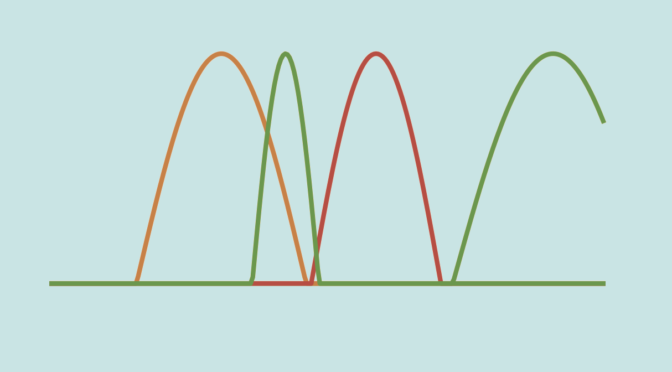# Counterexamples around Lebesgue’s Dominated Convergence Theorem

Let’s recall Lebesgue’s Dominated Convergence Theorem. Let $$(f_n)$$ be a sequence of real-valued measurable functions on a measure space $$(X, \Sigma, \mu)$$. Suppose that the sequence converges pointwise to a function $$f$$ and is dominated by some integrable function $$g$$ in the sense that $\vert f_n(x) \vert \le g (x)$ for all $$n \in \mathbb N$$ and all $$x \in X$$.
Then $$f$$ is integrable and $\lim\limits_{n \to \infty} \int_X f_n(x) \ d \mu = \int_X f(x) \ d \mu$

### Let’s see what can happen if we drop the domination condition.

We consider the space $$\mathbb R$$ endowed with Lebesgue measure and for $$E \subseteq \mathbb R$$ we denote by $$\chi_E$$ the indicator function of $$E$$ defined by $\chi_E(x)=\begin{cases} 1 \text{ if } x \in E\\ 0 \text{ otherwise}\end{cases}$ For $$n \in \mathbb N$$, the function $$f_n=\frac{1}{2n}\chi_{(n^2-n,n^2+n)}$$ is measurable and we have $\int_{\mathbb R} \frac{1}{2n}\chi_{(n^2-n,n^2+n)}(x) \ dx = \int_{n^2-n}^{n^2+n} \frac{1}{2n} \ dx = 1$ The sequence $$(f_n)$$ converges uniformly (and therefore pointwise) to the always vanishing function as for $$n \in \mathbb N$$ we have for all $$x \in \mathbb R$$ $$\vert f_n(x) \vert \le \frac{1}{2n}$$. Hence the conclusion of Lebesgue’s Dominated Convergence Theorem doesn’t hold for the sequence $$(f_n)$$.

Let’s verify that the sequence $$(f_n)$$ is not dominated by some integrable function $$g$$. For $$p < q$$ integers, we have \begin{aligned} q^2-q-(p^2+p) &= q^2-p^2 -q-p\\ &= (q-p)(q+p) -q -p\\ &\ge (q+p) -q-p=0 \end{aligned} Hence for $$p \neq q$$ integers the intervals $$(p^2-p,p^2+p)$$ and $$(q^2-q,q^2+q)$$ are disjoint. Consequently for all $$x \in \mathbb R$$ the sum $$\sum_{n \in \mathbb N} f_n(x)$$ amounts to only one term and the function $$\sum_{n \in \mathbb N} f_n$$ is well defined. If $$g$$ dominates the sequence $$(f_n)$$, it satisfies $$0 \le \sum_{n \in \mathbb N} f_n \le g$$. But $\int_{\mathbb R} \sum_{n \in \mathbb N} f_n(x) \ dx = \sum_{n \in \mathbb N} \int_{\mathbb R} f_n(x) \ dx = \sum_{n \in \mathbb N} 1 = \infty$ and $$g$$ cannot be integrable.

### The case of a nowhere converging function

Let’s now construct a sequence of continuous functions $$g_n$$ defined on $$[0, 1]$$ such that $$0 \le g_n \le 1$$ and $\lim\limits_{n \to \infty} \int_0^1 g_n(x) \ dx = 0,$ but such that the sequence $$(g_n(x))$$ converges for no $$x \in [0,1]$$.

Consider first the continuous and non negative function $$c : \mathbb R \to [0,1]$$ defined by $c(x) = \begin{cases} \cos \frac{\pi x}{2} &\text{for } x \in [-1,1]\\ 0 &\text{otherwise} \end{cases}$ We have $\int_{\mathbb R} c(x) \ dx = \int_{-1}^1 \cos \frac{\pi x}{2} \ dx = \frac{4}{\pi}$

Let’s also denote by $$(r_n)$$ the sequence whose initial terms are $\frac{1}{2}, \frac{1}{3}, \frac{2}{3}, \frac{1}{4}, \frac{2}{4}, \frac{3}{4}, \frac{1}{5}, \frac{2}{5}, \frac{3}{5}, \frac{4}{5}, \frac{1}{6}, \frac{2}{6}, \frac{3}{6}, \frac{4}{6}, \frac{5}{6}, \dots$ and whose formal definition is $r_n=\begin{cases} \frac{1}{2} &\text{ for } n= 1\\ \frac{n}{k+2} – \frac{k(k+1)}{2(k+2)} &\text{ for } \frac{k(k+1)}{2} < n \le \frac{(k+1)(k+2)}{2} \end{cases}$ We already encountered that sequence here. We define $$g_n : [0,1] \to [0,1]$$ by $g_n(x)=\begin{cases} c(x) &\text{ for } n= 1\\ c \left(\frac{k+2}{2}(x-r_n) \right) &\text{ for } \frac{k(k+1)}{2} < n \le \frac{(k+1)(k+2)}{2} \end{cases}$ All the $$g_n$$ functions are continuous because $$c$$ is continuous and we have for $$n > 1$$$0 \le \int_0^1 g_n(x) \ dx \le \int_{\mathbb R} c \left(\frac{k+2}{2}(x-r_n) \right) \ dx = \frac{8}{\pi (k+2)}$ Hence $\lim\limits_{n \to \infty} \int_0^1 g_n(x) \ dx = 0$ We’ll be done if we prove that for all $$x \in [0,1]$$, $$(g_n(x))$$ diverges. The detailed proof is left to the reader. The idea being for $$N \in \mathbb N$$ to find $$N < \frac{k(k+1)}{2} < n_1 \le \frac{(k+1)(k+2)}{2}$$ such that $$g_{n_1}(x)=0$$ and $$N < \frac{k(k+1)}{2} < n_2 \le \frac{(k+1)(k+2)}{2}$$ such that $$g_{n_2}(x) \ge \frac{\sqrt 2}{2}$$.